Journal of Astronomy and Space Sciences
The Korean Space Science Society
Research Paper

# Investigating Exoplanet Orbital Evolution Around Binary Star Systems with Mass Loss

1Department of Astronomy and Space Science, Cairo University, Cairo 12613, Egypt
2Lille Laboratory of Astronomy, Lille University of Science and Technology, Lille 59000, France
Corresponding Authorrahoma@sci.cu.edu.eg+20-2-3567-6843, +20-2-3572-7566

Received: Nov 8, 2016; Revised: Nov 28, 2016; Accepted: Nov 30, 2016

## Abstract

A planet revolving around binary star system is a familiar system. Studies of these systems are important because they provide precise knowledge of planet formation and orbit evolution. In this study, a method to determine the evolution of an exoplanet revolving around a binary star system using different rates of stellar mass loss will be introduced. Using a hierarchical triple body system, in which the outer body can be moved with the center of mass of the inner binary star as a two-body problem, the long period evolution of the exoplanet orbit is determined depending on a Hamiltonian formulation. The model is simulated by numerical integrations of the Hamiltonian equations for the system over a long time. As a conclusion, the behavior of the planet orbital elements is quite affected by the rate of the mass loss from the accompanying binary star.

Keywords: exoplanet; long period dynamics; hierarchical system; triple star systems

## 1 INTRODUCTION

In the last two decades, studies related to exoplanets have become an important field in modern astrophysics, as observational instrumentations have become more sensitive and efficient for detecting signals related to this type of planet. While there are more than 100 cataloged exoplanets orbiting single star, the studies of such planets, including detection, characterization, and evolution, are still difficult. In addition, recent discoveries confirm the presence of an exoplanet around a binary companion (Borucki et al. 2010). Therefore, many stars in the solar neighborhood are a member of binary or multiple systems, which will force us to modify our knowledge regarding the dynamics of the planets in binary star systems. The dynamics of planets in binary systems are not easy to formulate because of the second star perturbations, which require researchers to neglect other perturbations like star radiation. In a hierarchical triple system, the motion of a planet will be constrained to revolve around the center of the binary star as a two-body problem.

Systems with multiple stars and planetary components draw the attention of researchers with their rich dynamics that can carry information about planetary formation and evolution.

Raghavan et al. (2010) found approximately half of the stars in the Sun neighborhood in multiple systems. At about a few tens of AU, these local systems have planets like those hosted by single stars, presenting interactions between stellar and planetary dynamics. Marzari et al. (2005) focused on the planetary systems subjected to planet–planet scattering around a binary star system. They studied the orbits of the unejected planets. Triple-body systems in which a planet revolves around a binary star system can be treated as hierarchical stable systems, and the motion can be described by two related systems. Each system describes a two-body problem: the motion of the inner binary stars and the motion of the planet with the center of mass of the inner system. The hierarchical system dynamics, especially the long period or secular terms, attracted the attention of researchers such as Harrington (1968), Heppenheimer (1978), Ford et al. (2000), Lee & Peale (2003), Farago & Laskar (2010), Leung & Lee (2013), Naoz et al. (2013a, 2013b), Li et al. (2014), and Liu et al. (2015). As any gravitational perturbations in the binary system will strongly affect the motion of the planet, many studies introduced the dynamic stability of planets, especially the perturbation due to a secondary star (Boss 2006; Haghighipour 2006; Kley & Nelson 2008; Thébault et al. 2009; Giuppone et al. 2011). Carvalho et al. (2016) introduced triple system secular dynamics assuming a center star like the Sun and a planet like Jupiter all perturbed by another brown dwarf star.

In this work, the long period dynamics of the exoplanet will be formulated taking into consideration different rates of mass loss from the second inner companion using a hierarchical triple system to formulate the equations.

## 2 PROBLEM MODEL

In the computations, we utilized a triple body system where an outer body or exoplanet of mass, m2, orbited the center of mass of two inner bodies or binary stars of mass (m0 and m1). Each system was described by two perturbed Keplerian bodies.

Using Jacobi coordinates, ${\stackrel{\to }{r}}_{1}$ and ${\stackrel{\to }{r}}_{2}$ is the position vector of m1 to m0, and ${\stackrel{\to }{r}}_{2}$ is the position vector of m2 to the center of mass of the binary stars, respectively (see Fig. 1). In addition to well-known Keplerian elements, the semi-major axis aj, the eccentricity ej, the inclination ij, the right ascension of the ascending node Ωj, the argument of periastron ωj, and the mean anomaly Mj, the true anomaly fj is used, where j=1, 2 for the inner orbit (j=1) and outer orbit (j=2).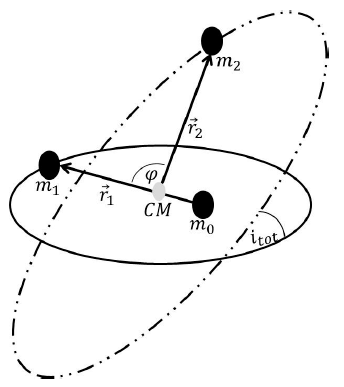Fig. 1. Exoplanet orbit considering a triple body system.

The Hamiltonian of the system is determined as (Harrington 1968; Ford et al. 2000, 2004; Takeda et al. 2008):(1)

$\begin{array}{c}H=\frac{g{m}_{1}{m}_{2}}{2{a}_{1}}+\frac{g{m}_{3}\left({m}_{1}+{m}_{2}\right)}{2{a}_{2}}\\ +\frac{g}{{a}_{2}}\sum _{s\ge 2}{\alpha }^{s}{M}_{s}{\left(\frac{{r}_{1}}{{a}_{1}}\right)}^{2}{\left(\frac{{a}_{2}}{{r}_{2}}\right)}^{s+1}{P}_{s}\left(\mathrm{cos}\phi \right)\end{array}$
(1)

where g is the gravitational constant, α=a1/a2,p1 are Legendre polynomials, is the angle between r1 and r2, and

${M}_{s}={m}_{0}{m}_{1}{m}_{2}\frac{{m}_{0}^{s-1}-{\left(-{m}_{1}\right)}^{s-1}}{{\left({m}_{0}+{m}_{1}\right)}^{s}}$

The Delaunay’s elements are defined as: lj is the mean of the anomalies of object, the arguments of periastron, gj and the longitudes of the ascending nodes, hj and conjugate momenta,

The Hamiltonian is given by Michaely & Perets (2014) as:

$H={H}_{0}+{H}_{1}+{H}_{2}$
(2)

where

$\begin{array}{c}{H}_{0}=-\frac{{\beta }_{0}}{2{L}_{1}^{2}}-\frac{{\beta }_{0}}{2{L}_{2}^{2}}\\ {H}_{1}=-4{\beta }_{2}\left(\frac{{L}_{1}^{4}}{{L}_{2}^{6}}\right){\left(\frac{{r}_{1}}{{a}_{1}}\right)}^{2}{\left(\frac{{a}_{2}}{{r}_{2}}\right)}^{3}\left(3{\text{cos}}^{2}\phi -1\right)\\ {H}_{2}=-2{\beta }_{3}\left(\frac{{L}_{1}^{6}}{{L}_{2}^{8}}\right){\left(\frac{{r}_{1}}{{a}_{1}}\right)}^{3}{\left(\frac{{a}_{2}}{{r}_{2}}\right)}^{4}\left(5{\text{cos}}^{3}\phi -3\text{cos}\phi \right)\end{array}$

with

$\begin{array}{l}{\beta }_{0}=g{m}_{0}{m}_{1}\frac{{L}_{0}^{2}}{{a}_{1}}\\ {\beta }_{1}=g{m}_{2}\left({m}_{0}+{m}_{1}\right)\frac{{L}_{2}^{2}}{{a}_{2}}\\ {\beta }_{2}=\frac{g}{16}\frac{{\left({m}_{0}+{m}_{1}\right)}^{7}{m}_{2}^{7}}{16{\left({m}_{0}{m}_{1}\right)}^{3}{\left({m}_{0}+{m}_{1}+{m}_{2}\right)}^{3}}\\ {\beta }_{3}=\frac{g}{4}\frac{{\left({m}_{0}+{m}_{1}\right)}^{9}{m}_{2}^{9}\left({m}_{0}-{m}_{1}\right)}{4{\left({m}_{0}{m}_{1}\right)}^{5}{\left({m}_{0}+{M}_{1}+{m}_{2}\right)}^{4}}\end{array}$

and cosφ can be written using orbital elements as in Rahoma (2014) and Rahoma & Deleflie (2014)(3)

$\text{cos\hspace{0.17em}}\phi =\sum _{i,\text{\hspace{0.17em}}j=-1}^{1}{A}_{ij}\text{cos}\left({f}_{1}+{\omega }_{1}+i\left({f}_{2}+{\omega }_{2}\right)+j\left({\Omega }_{2}-{\Omega }_{1}\right)\right)$
(3)

where the non-vanishing coefficients are

$\begin{array}{l}{A}_{-1-1}=\frac{1}{4}\left(1+\text{cos\hspace{0.17em}}{i}_{1}+\text{cos\hspace{0.17em}}{i}_{2}+\mathrm{cos }{i}_{1}\text{\hspace{0.17em}cos\hspace{0.17em}}{i}_{2}\right)\\ {A}_{-10}=\frac{1}{2}\text{sin\hspace{0.17em}}{i}_{1}\text{\hspace{0.17em}sin\hspace{0.17em}}{i}_{2}\\ {A}_{-11}=\frac{1}{4}\left(1-\text{cos\hspace{0.17em}}{i}_{1}-\text{cos\hspace{0.17em}}{i}_{2}+\text{cos\hspace{0.17em}}{i}_{1}\text{\hspace{0.17em}cos\hspace{0.17em}}{i}_{2}\right)\\ {A}_{1-1}=\frac{1}{4}\left(1+\text{cos\hspace{0.17em}}{i}_{1}-\text{cos\hspace{0.17em}}{i}_{2}-\text{cos}{i}_{1}\text{cos}{i}_{2}\right)\\ {A}_{10}=-\frac{1}{2}\text{sin\hspace{0.17em}}{i}_{1}\text{\hspace{0.17em}sin\hspace{0.17em}}{i}_{2}\\ {A}_{11}=\frac{1}{4}\left(1-\mathrm{cos}\text{\hspace{0.17em}}{i}_{1}+\mathrm{cos}\text{\hspace{0.17em}}{i}_{2}-\mathrm{cos}\text{\hspace{0.17em}}{i}_{1}\text{\hspace{0.17em}}\mathrm{cos}{i}_{2}\right)\end{array}$

## 3 CALCULATIONS OF THE LONG TERMS DYNAMICS

In most cases, it is better to present the movement over the long term, especially when discussing exoplanets. Therefore, the short period terms must be excluded by averaging the Hamiltonian (Eq. (2)) of the system over l1 and l2, where the conjugate momenta, L1 and L1, were included in the unperturbed terms to provide a smooth motion over the long period .

The standard average definition is $\stackrel{˜}{Q}=\frac{1}{2\pi }{\int }_{0}^{2\pi }Q\text{\hspace{0.17em}}dl$, and the normalized Hamiltonian is given as(4)(5)(6)

$\stackrel{˜}{H}={\stackrel{˜}{H}}_{0}+{\stackrel{˜}{H}}_{1}+{\stackrel{˜}{H}}_{2}$
(4)

where

${\stackrel{˜}{H}}_{0}=\frac{{\beta }_{1}}{2{L}_{1}^{2}}+\frac{{\beta }_{2}}{2{L}_{2}^{2}}$
(5)

$\begin{array}{c}{\stackrel{˜}{H}}_{1}=-4{\beta }_{2}\left(\frac{{L}_{1}^{4}}{{L}_{2}^{6}}\right)\\ ×\sum _{j,\text{\hspace{0.17em}}k=-2}^{2}\left\{{\alpha }_{jk}\left({e}_{1},\text{\hspace{0.17em}}{e}_{2}\right){\beta }_{jk}\left({i}_{1},\text{\hspace{0.17em}}{i}_{2}\right)\text{\hspace{0.17em}cos}\left(j{g}_{1}+k\left({h}_{2}-{h}_{1}\right)\right)\right\}\end{array}$
(6)

and the non-vanishing coefficients are(7)

$\begin{array}{l}{\alpha }_{00}={\alpha }_{01}={\alpha }_{02}=\frac{\left(2+3{e}_{1}^{2}\right)}{2{\left(1-{e}_{2}^{2}\right)}^{3/2}}\\ {\alpha }_{20}={\alpha }_{2-2}={\alpha }_{2-1}={\alpha }_{21}={\alpha }_{22}=\frac{5{e}_{1}^{2}}{2{\left(1-{e}_{2}^{2}\right)}^{3/2}}\\ {\beta }_{00}=-1+\frac{3}{2}\left({A}_{-1-1}^{2}+{A}_{-10}^{2}+{A}_{-11}^{2}+{A}_{1-1}^{2}+{A}_{10}^{2}+{A}_{11}^{2}\right)\\ {\beta }_{01}=3\left({A}_{-1-1}{A}_{-10}+{A}_{-10}{A}_{-11}+{A}_{1-1}{A}_{10}+{A}_{10}{A}_{11}\right)\\ {\beta }_{02}=\left(3{A}_{-1-1}{A}_{-11}+{A}_{1-1}{A}_{11}\right)\\ {\beta }_{20}=3\left({A}_{-11}{A}_{1-1}+{A}_{-10}{A}_{10}+{A}_{-1-1}{A}_{11}\right)\\ {\beta }_{2-2}=3\left({A}_{-1-1}{A}_{1-1}\right)\\ {\beta }_{2-1}=3\left({A}_{-10}{A}_{1-1}+{A}_{-1-1}{A}_{10}\right)\\ {\beta }_{21}=3\left({A}_{-11}{A}_{10}+{A}_{-10}{A}_{11}\right)\\ {\beta }_{22}=3\left({A}_{-11}{A}_{11}\right)\\ {\stackrel{˜}{H}}_{2}=-2{\beta }_{3}\left(\frac{{L}_{1}^{6}}{{L}_{2}^{8}}\right)\\ \text{ }×\sum _{j,s,k=-3}^{3}\left\{{\alpha }_{jsk}\left({e}_{1},\text{\hspace{0.17em}}{e}_{2}\right){\beta }_{jsk}\left({i}_{1},\text{\hspace{0.17em}}{i}_{2}\right)\text{\hspace{0.17em}cos}\left(j{g}_{1}+s{g}_{2}+k\left({h}_{2}-{h}_{1}\right)\right)\right\}\end{array}$
(7)

The non-vanishing coefficients ${\alpha }_{jsk}\left({e}_{1},\text{\hspace{0.17em}}{e}_{2}\right)$ and ${\beta }_{jsk}\left({i}_{1},\text{\hspace{0.17em}}{i}_{2}\right)$ can be found in Appendix I.

Now the equations of motion can be written by using the Hamiltonian equations. For a system (qi, Qi), qi are the coordinates and Qi are the momenta with Hamiltonian F(qi, Qi, t).

The equations of motion can be derived as:(8)

${\stackrel{˙}{q}}_{i}=\frac{d{q}_{i}}{dt}=\frac{\partial F}{\partial {Q}_{i}},\text{ }{\stackrel{˙}{Q}}_{i}=\frac{d{Q}_{i}}{dt}=-\frac{\partial F}{\partial {q}_{i}}$
(8)

where t reffered to the time

## 4 STAR MASS LOSS

The key process in star studies, generally and especially for massive stars, is the mass loss; that is, the slow change in the mass of the star with respect to its orbital period. This must be understood well along with its effect on the surrounding environment. This will then guarantee complete success in astrophysical applications. All stars are undergoing mass loss with the amount depending on the star's size and its evolution stage. For instance, the Sun is losing 7 billion tons per hour, while supergiant stars emit a larger amount of energy.

In this study, the long period dynamics with mass loss will be treated by assuming the mass loss occurs isotropically. In addition, the mass loss rate will be varied depending on stellar evolutionary stage and the mechanism of mass loss. For more information about mass loss refer to Rahoma et al. (2009) and the references therein.

To simplify the dynamics, the mass loss from mass m will be assumed to be of the form(9)

$\frac{d}{dt}m=\alpha$
(9)

where α is a constant.

## 5 NUMERICAL SIMULATIONS

In this section, the simulated effect of mass loss on the exoplanet is described. The second companion in the binary star system is chosen to loss mass over time at different rates. The orbital element variations constructed from the Hamiltonian equations are investigated numerically.

We suppose that a hierarchical triple system has the intiial condition of a1=3 AU, e1=0.23, i1=67°, ω1=179° and Ω1=120° and a2=40 AU, e2=0.5, i2=45°, ω2=120° and Ω2=15°, where the mass of m0 and m1 are chosen as 15 and 5 times the mass of the Sun, respectively, and the mass of the planet, m2, is 0.01 M. The integration is run over a long period of 5 × 105 years.

## 6 RESULTS, COMMENTS AND DISCUSSION

Figs. 2-6 show the variation of the orbital elements as a function of time for a planet orbiting a binary star system in which the second companion member loses mass. The initial conditions were chosen empirically. Smooth curves were presented as expected; therefore, the average technique is used to eliminate the short period terms.Fig. 3Fig. 4Fig. 5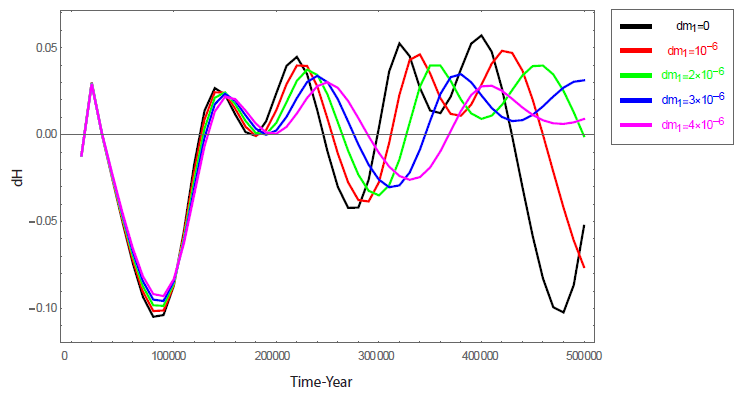Fig. 2. Evolution of exoplanet orbital elements dH within a triple system framework with mass loss from the secondary inner companion with different values per year.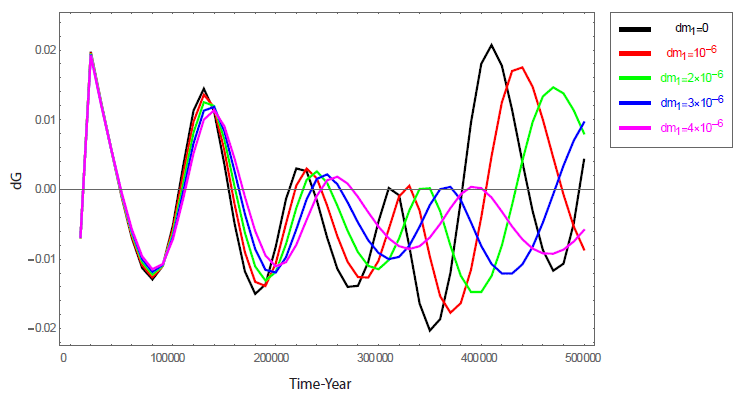Fig. 3. Evolution of exoplanet orbital elements dG within a triple system framework with mass loss from the secondary inner companion with different values per year.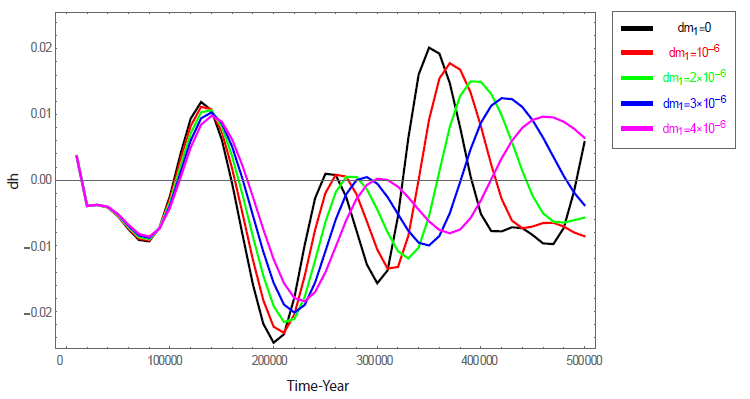Fig. 4. Evolution of exoplanet orbital elements dh within a triple system framework with mass loss from the secondary inner companion with different values per year.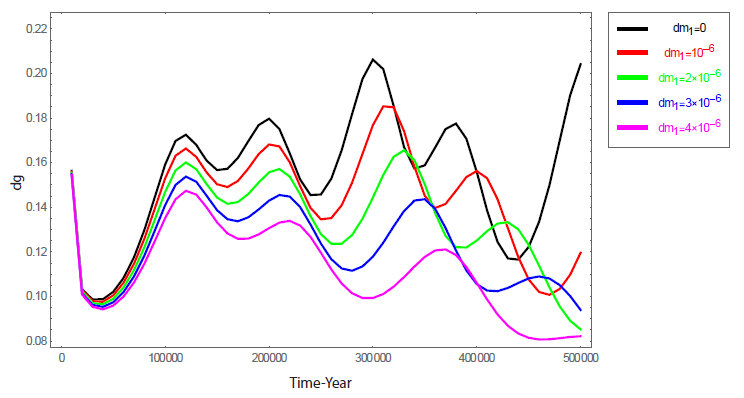Fig. 5. Evolution of exoplanet orbital elements dg within a triple system framework with mass loss from the secondary inner companion with different values per year.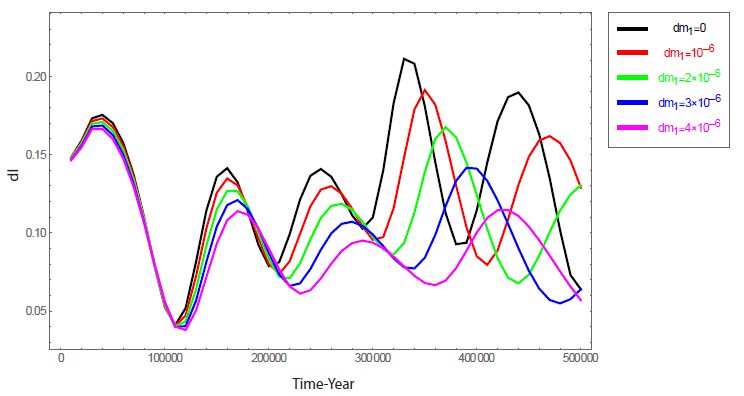Fig. 6. Evolution of exoplanet orbital elements dl within a triple system framework with mass loss from the secondary inner companion with different values per year.

In Figs. 2-6, the orbital element evaluation is presented where the black line represents the case with no mass loss, the red line represents the case with mass loss = 10-6 M/yr, the green line represents the case with mass loss = 2 × 10-6 M/yr, the blue line represents the case with mass loss = 3 × 10-6 M/yr, and the fuchsia line represents the case with mass loss = 4 × 10-6 M/yr. It is noted that: 1) as the value of mass loss increases, the behavior of the orbital element evolution changes and becomes distant from the behavior of the black line; 2) even if all behaviors are periodic, the amplitude changes with the value of mass loss; 3) in the first approximately 15 to 20 × 104 years, all behaviors nearly coincide; but after that time, the behaviors are completely different; and 4) in all figures, the secular term is imposed on the long period terms, where there is a slope in the curves.

## 7 CONCLUSIONS

Supported by observations, many exoplanets are orbiting binary star systems, with nearly 70 exoplanets found in binary stellar systems (Schwarz et al. 2015). This work studied the effects of stellar mass loss from one star in the inner binary system on its companion planetary orbit. It was found that the mass loss causes the orbit of the planet to spiral outward.

Using triple hierarchical system dynamics, the Hamiltonian of the system was constructed up to the second order with the relative semimajor axis of the binary star to the semimajor axis of the planet as a small parameter. Previous work regarding the effect of stellar mass loss on planetary orbits showed that the semimajor axis and mass production remained nearly constant (Veras et al. 2011; Mustill & Villaver 2012) unlike the results of this study, which adopted mass changes with time. The behavior of the orbital elements over a long time with different rates of mass loss was presented. Smooth curves over a long time resulted; As the short period terms are averaged. The trajectory presented an oscillatory behavior along the time where the oscillation period amplitude decreased with an increasing value of the outer companion mass loss. The accuracy could be improved by raising the order of expansion. The mass change models could be imposed on the equations.

## ACKNOWLEDGMENTS

This work was supported by the French and Egyptian Governments through a co-financed fellowship granted by the French Embassy in Egypt (Institut Francais d'Egypte) and the Science and Technology Development Fund (STDF).

## References

1.

Borucki WJ, Koch D, Basri G, Batalha N, Brown T , et al. Kepler planet-detection mission introduction and first results. Science. 2010; 327:977-980.2.

Boss AP. Gas giant protoplanets formed by disk instability in binary star systems. Astrophys. J. 2006; 641:1148-1161.3.

Carvalho JPS, Mourão DC, de Moraes RV, Prado AFBA, Winter OC. Exoplanets in binary star systems on the switch from prograde to retrograde orbits. Celest. Mech. Dyn. Astron. 2016; 124:73-96.4.

Farago F, Laskar J. High-inclination orbits in the secular quadrupolar three-body problem. Mon. Not. Roy. Astron. Soc. 2010; 401:1189-1198.5.

Ford EB, Kozinsky B, Rasio FA. Secular evolution of hierarchical triple star systems. Astrophys. J. 2000; 535:385-401.6.

Ford EB, Kozinsky B, Rasio FA. Erratum “secular evolution of hierarchical triple star systems”. (ApJ 535 385:). Astrophys. J. 2004; 605:966-966.7.

Giuppone CA, Leiva AM, Correa-Otto J, Beaugé C. Secular dynamics of planetesimals in tight binary systems application to γ-Cephei. Astron. Astrophys. 2011; 530:A103.8.

Haghighipour N. Dynamical stability and habitability of the γ Cephei binary-planetary system. Astrophys. J. 2006; 644:543-550.9.

Harrington RS. Dynamical evolution of triple stars. Astron. J. 1968; 73:190-194.10.

Heppenheimer TA. On the formation of planets in binary star systems. Astron. Astrophys. 1978; 65:421-426.11.

Kley W, Nelson RP. Planet formation in binary stars the case of γ Cephei. Astron. Astrophys. 2008; 486:617-628.12.

Lee MH, Peale SJ. Secular evolution of hierarchical planetary systems. Astrophys. J. 2003; 592:1201-1216.13.

Leung GCK, Lee MH. An analytic theory for the orbits of circumbinary planets. Astrophys. J. 2013; 763:107-119.14.

Li G, Naoz S, Holman M, Loeb A. Chaos in the test particle eccentric Kozai-Lidov mechanism. Astrophys. J. 2014; 791:86.15.

Liu B, Muñoz DJ, Lai D. Suppression of extreme orbital evolution in triple systems with short-range forces. Mon. Not. Roy. Astron. Soc. 2015; 447:747-764.16.

Marzari F, Weidenschilling SJ, Barbieri M, Granata V. Jumping Jupiters in binary star systems. Astrophys. J. 2005; 618:502-511.17.

Michaely E, Perets HB. Secular dynamics in hierarchical threebody systems with mass loss and mass transfer. Astrophys. J. 2014; 794:122.18.

Mustill AJ, Villaver E. Foretellings of Ragnarök world-engulfing asymptotic giants and the inheritance of white dwarfs. Astrophys. J. 2012; 761:121.19.

Naoz S, Farr WM, Lithwick Y, Rasio FA, Teyssandier J. Secular dynamics in hierarchical three-body systems. Mon. Not. Roy. Astron. Soc. 2013a; 431:2155-2171.20.

Naoz S, Kocsis B, Loeb A, Yunes N. Resonant post-Newtonian eccentricity excitation in hierarchical three-body systems. Astrophys. J. 2013b; 773:187.21.

Raghavan D, McAlister HA, Henry TJ, Latham DW, Marcy GW , et al. A survey of stellar families multiplicity of solartype stars. Astrophys. J. 2010; 190:1-42.22.

Rahoma WA. Orbital elements evolution due to a perturbing body in an inclined elliptical orbit. J. Astron. Space Sci. 2014; 31:199-204.23.

Rahoma WA, Deleflie F. Effects of J2 perturbation on geometrical relative motion, Indian. J. Sci. Tech. 2014; 7:968-974.24.

Rahoma WA, Abd El-Salam FA, Ahmed MK. Analytical treatment of the two-body problem with slowly varying mass. J. Astrophys. Astron. 2009; 30:187-205.25.

Schwarz R, Funk B, Bazsó . On the possibility of habitable Trojan planets in binary star systems. Orig. Life Evol. Biosph. 2015; 45:469-477.26.

Takeda G, Kita R, Rasio FA. Planetary systems in binaries I dynamical classification. Astrophys. J. 2008; 683:1063-1075.27.

Thébault P, Marzari F, Scholl H. Planet formation in the habitable zone of α Centauri B. Mon. Not. Roy. Astron. Soc. Lett. 2009; 393:L21-L25.28.

Veras D, Wyatt MC, Mustill AJ, Bonsor A, Eldridge JJ. The great escape how exoplanets and smaller bodies desert dyingstars. Mon. Not. Roy. Astron. Soc. 2011; 417:2104-2123.## Appendices

Appendix
Appendix I

The non-vanishing coefficients of Eq. (6).

$\begin{array}{c}{\alpha }_{-3-11}={\alpha }_{-3-12}={\alpha }_{-3-13}={\alpha }_{3-10}={\alpha }_{3-11}={\alpha }_{3-12}\\ ={\alpha }_{3-13}={\alpha }_{-311}={\alpha }_{-312}={\alpha }_{-313}={\alpha }_{310}\\ ={\alpha }_{311}={\alpha }_{312}={\alpha }_{313}=\frac{-35{e}_{1}^{2}\left(2-3{e}_{2}^{2}\right)}{16{\left(1-{e}_{2}^{2}\right)}^{3/2}}\end{array}$

$\begin{array}{c}{\alpha }_{-1-11}={\alpha }_{-1-12}={\alpha }_{-1-13}={\alpha }_{1-10}={\alpha }_{1-11}={\alpha }_{1-12}\\ ={\alpha }_{1-13}={\alpha }_{-111}={\alpha }_{-112}={\alpha }_{-113}={\alpha }_{110}={\alpha }_{111}\\ ={\alpha }_{112}={\alpha }_{113}=\frac{-5\left(2-3{e}_{2}^{2}\right)\left(3+4{e}_{1}^{2}\right)}{16{\left(1-{e}_{2}^{2}\right)}^{3/2}}\end{array}$

${\beta }_{-3-13}=\frac{15}{4}\left({A}_{1-1}^{2}{A}_{-1-1}\right)$

${\beta }_{-3-12}=\frac{15}{4}\left({A}_{1-1}^{2}{A}_{-10}+2{A}_{-1-1}{A}_{1-1}{A}_{10}\right)$

$\begin{array}{c}{\beta }_{-3-11}=\frac{15}{4}\left({A}_{1-1}^{2}{A}_{-11}+{A}_{10}^{2}{A}_{-1-1}\\ +2\left({A}_{-10}{A}_{1-1}{A}_{10}+{A}_{-1-1}{A}_{1-1}{A}_{11}\right)\right)\end{array}$

$\begin{array}{c}{\beta }_{-311}=\frac{15}{4}\left({A}_{-10}^{2}{A}_{1-1}+{A}_{-1-1}^{2}{A}_{11}\\ +2\left({A}_{-1-1}{A}_{-11}{A}_{1-1}+{A}_{-1-1}{A}_{-10}{A}_{10}\right)\right)\end{array}$

$\begin{array}{l}{\beta }_{-312}=\frac{15}{4}\left({A}_{-1-1}^{2}{A}_{10}+2{A}_{-1-1}{A}_{-10}{A}_{1-1}\right)\\ {\beta }_{-313}=\frac{15}{4}\left({A}_{-1-1}^{2}{A}_{1-1}\right)\\ {\beta }_{3-13}=\frac{15}{4}\left({A}_{-11}^{2}{A}_{11}\right)\\ {\beta }_{3-12}=\frac{15}{4}\left({A}_{-11}^{2}{A}_{-10}+2{A}_{-10}{A}_{-11}{A}_{11}\right)\end{array}$

$\begin{array}{c}{\beta }_{3-11}=\frac{15}{4}\left({A}_{-11}^{2}{A}_{1-1}+2{A}_{-10}^{2}{A}_{11}\\ +2\left({A}_{-10}{A}_{-11}{A}_{10}+{A}_{-1-1}{A}_{-11}{A}_{11}\right)\right)\end{array}$

$\begin{array}{c}{\beta }_{3-10}=\frac{15}{4}\left({A}_{-10}^{2}{A}_{10}\\ +2\left({A}_{-10}{A}_{-11}{A}_{1-1}+{A}_{-1-1}{A}_{-11}{A}_{10}\\ +{A}_{-1-1}{A}_{-10}{A}_{11}\right)\right)\end{array}$

$\begin{array}{c}{\beta }_{310}=\frac{15}{4}\left({A}_{10}^{2}{A}_{-10}\\ +2\left({A}_{-11}{A}_{1-1}{A}_{10}+{A}_{-10}{A}_{1-1}{A}_{11}\\ +{A}_{-1-1}{A}_{-10}{A}_{11}\right)\right)\end{array}$

$\begin{array}{c}{\beta }_{311}=\frac{15}{4}\left({A}_{10}^{2}{A}_{-11}+{A}_{11}^{2}{A}_{-1-1}\\ +2\left({A}_{-11}{A}_{1-1}{A}_{11}+{A}_{-10}{A}_{10}{A}_{11}\right)\right)\end{array}$

$\begin{array}{l}{\beta }_{312}=\frac{15}{4}\left({A}_{11}^{2}{A}_{-10}+2{A}_{-11}{A}_{10}{A}_{11}\right)\\ {\beta }_{313}=\frac{15}{4}\left({A}_{-11}^{2}{A}_{11}\right)\\ {\beta }_{-1-13}=\frac{15}{4}\left({A}_{1-1}^{2}{A}_{11}+2\left({A}_{-1-1}{A}_{-11}{A}_{1-1}\right)\right)\end{array}$

$\begin{array}{c}{\beta }_{-1-12}=\frac{15}{4}\left({A}_{1-1}^{2}{A}_{10}\\ +2\left({A}_{-1-1}{A}_{-10}{A}_{1-1}+{A}_{-10}{A}_{-11}{A}_{1-1}\\ +{A}_{-1-1}{A}_{-11}{A}_{10}+{A}_{1-1}{A}_{10}{A}_{11}\right)\right)\end{array}$

$\begin{array}{c}{\beta }_{-1-11}=-3{A}_{-11}+\frac{15}{4}\left({A}_{1-1}^{3}+{A}_{10}^{2}{A}_{11}\\ +2\left({A}_{-1-1}^{2}{A}_{1-1}+{A}_{-10}^{2}{A}_{1-1}+{A}_{10}^{2}{A}_{1-1}\\ +{A}_{11}^{2}{A}_{1-1}+{A}_{-1-1}{A}_{-11}{A}_{11}\right)\right)\end{array}$

$\begin{array}{c}{\beta }_{-111}=-3{A}_{-1-1}+\frac{15}{4}\left({A}_{-1-1}^{3}+{A}_{-10}^{2}{A}_{-11}\\ +2\left({A}_{-1-1}^{2}{A}_{-10}+{A}_{-11}^{2}{A}_{-1-1}\\ +{A}_{-1-1}^{2}{A}_{1-1}+{A}_{10}^{2}{A}_{-1-1}+{A}_{-1-1}^{2}{A}_{11}\\ +{A}_{-10}{A}_{1-1}{A}_{10}+{A}_{-11}{A}_{1-1}{A}_{11}+{A}_{-10}{A}_{10}{A}_{11}\right)\right)\end{array}$

$\begin{array}{c}{\beta }_{-112}=\frac{15}{4}\left({A}_{-1-1}^{2}{A}_{-10}\\ +2\left({A}_{-1-1}{A}_{-10}{A}_{-11}+{A}_{-1-1}{A}_{1-1}{A}_{10}\\ +{A}_{-10}{A}_{1-1}{A}_{11}+{A}_{-1-1}{A}_{10}{A}_{11}\right)\right)\end{array}$

$\begin{array}{l}{\beta }_{-113}=\frac{15}{4}\left({A}_{-1-1}^{2}{A}_{-11}+2\left({A}_{-1-1}{A}_{1-1}{A}_{11}\right)\right)\\ {\beta }_{1-13}=\frac{15}{4}\left({A}_{-11}^{2}{A}_{-1-1}+2\left({A}_{-11}{A}_{1-1}{A}_{11}\right)\right)\end{array}$

$\begin{array}{c}{\beta }_{1-12}=\frac{15}{4}\left({A}_{-11}^{2}{A}_{-10}+2\left({A}_{-1-1}{A}_{-10}{A}_{-11}+{A}_{-11}{A}_{1-1}{A}_{10}\right)\\ +{A}_{-10}{A}_{1-1}{A}_{11}+{A}_{-11}{A}_{10}{A}_{11}\right)\\ {\beta }_{1-13}=\frac{15}{4}\left({A}_{-11}^{2}{A}_{-1-1}+2\left({A}_{-11}{A}_{1-1}{A}_{11}\right)\right)\end{array}$

$\begin{array}{c}{\beta }_{1-11}=-3{A}_{-11}+\frac{15}{4}\left({A}_{-11}^{3}+{A}_{-10}^{2}{A}_{-1-1}\\ +2\left({A}_{-1-1}^{2}{A}_{-11}+{A}_{-10}^{2}{A}_{-11}+{A}_{-10}^{2}{A}_{-11}\\ +{A}_{10}^{2}{A}_{-11}+{A}_{11}^{2}{A}_{-10}+{A}_{1-1}{A}_{-10}{A}_{10}\\ +{A}_{-1-1}{A}_{1-1}{A}_{11}+{A}_{-10}{A}_{10}{A}_{11}\right)\end{array}$

$\begin{array}{c}{\beta }_{1-10}=-3{A}_{-10}+\frac{15}{4}\left({A}_{-10}^{3}\\ +2\left({A}_{-1-1}^{2}{A}_{-10}+{A}_{-11}^{2}{A}_{-10}+{A}_{1-1}^{2}{A}_{-10}\\ +{A}_{10}^{2}{A}_{-10}+{A}_{11}^{2}{A}_{-10}+{A}_{-1-1}{A}_{-10}{A}_{11}\\ +{A}_{-1-1}{A}_{1-1}{A}_{10}+{A}_{-11}{A}_{1-1}{A}_{10}\\ +{A}_{-1-1}{A}_{10}{A}_{11}+{A}_{-11}{A}_{10}{A}_{11}\right)\right)\end{array}$

$\begin{array}{c}{\beta }_{110}=-3{A}_{-10}+\frac{15}{4}\left({A}_{10}^{3}\\ +2\left({A}_{-1-1}^{2}{A}_{10}+{A}_{-10}^{2}{A}_{10}+{A}_{-11}^{2}{A}_{10}\\ +{A}_{1-1}^{2}{A}_{10}+{A}_{11}^{2}{A}_{10}+{A}_{-1-1}{A}_{-10}{A}_{1-1}\\ +{A}_{-10}{A}_{-11}{A}_{1-1}+{A}_{-1-1}{A}_{-10}{A}_{11}\\ +{A}_{-10}{A}_{-11}{A}_{11}+{A}_{1-1}{A}_{10}{A}_{11}\right)\right)\end{array}$

$\begin{array}{c}{\beta }_{111}=-3{A}_{11}+\frac{15}{4}\left({A}_{11}^{3}+{A}_{10}^{2}{A}_{1-1}\\ +2\left({A}_{-1-1}^{2}{A}_{11}+{A}_{-10}^{2}{A}_{11}+{A}_{-11}^{2}{A}_{11}\\ +{A}_{1-1}^{2}{A}_{11}+{A}_{10}^{2}{A}_{11}+{A}_{-1-1}{A}_{-11}{A}_{1-1}\\ +{A}_{-1-1}{A}_{-10}{A}_{10}+{A}_{-10}{A}_{-11}{A}_{10}\right)\right)\end{array}$

$\begin{array}{c}{\beta }_{112}=\frac{15}{4}\left({A}_{11}^{2}{A}_{10}+2\left({A}_{-1-1}{A}_{-11}{A}_{10}+{A}_{-1-1}{A}_{-10}{A}_{11}\\ +{A}_{-10}{A}_{-11}{A}_{11}+{A}_{1-1}{A}_{10}{A}_{11}\right)\right)\end{array}$

$\begin{array}{c}{\beta }_{113}=\frac{15}{4}\left({A}_{11}^{2}{A}_{1-1}+2\left({A}_{-1-1}{A}_{-11}{A}_{11}\right)\right)\\ +{A}_{-10}{A}_{-11}{A}_{11}+{A}_{1-1}{A}_{10}{A}_{11}\right)\right)\end{array}$